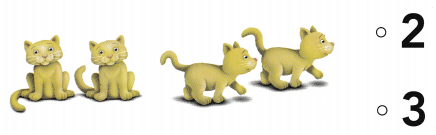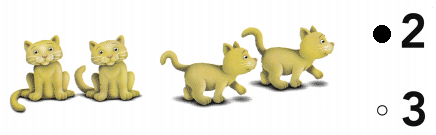# Texas Go Math Kindergarten Lesson 12.2 Answer Key Write Subtraction

Refer to our Texas Go Math Kindergarten Answer Key Pdf to score good marks in the exams. Test yourself by practicing the problems from Texas Go Math Kindergarten Lesson 12.2 Answer Key Write Subtraction.

## Texas Go Math Kindergarten Lesson 12.2 Answer Key Write Subtraction

Explore

DIRECTIONS: There are five fish. One fish swims away. How many fish are left? Trace the circle and X to show the fish swimming away. Trace the subtraction sentence.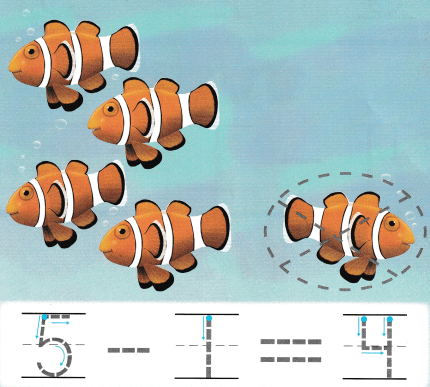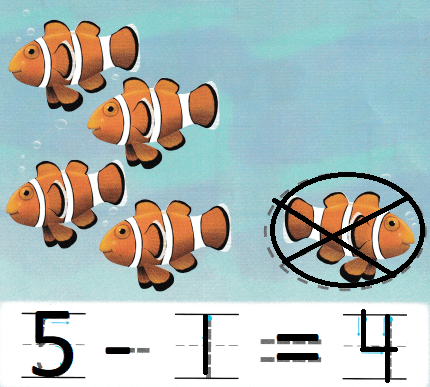Explanation:
There are five fish.
5 – 1 = 4
so, 4 fish are left

Share and Show

DIRECTIONS: 1. Listen to the subtraction word problem. Trace the circle and X to show how many crawl away. Trace to complete the subtraction sentence. 2-3. Listen to the subtraction word problem. Draw a circle and mark an X to show how many leave. Trace and write to complete the subtraction sentence.

Question 1.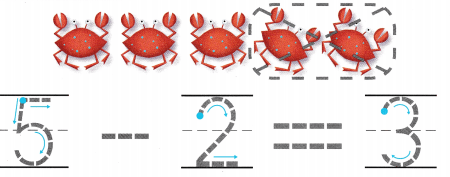Explanation:
There are 5 crabs
2 crabs crawl away
5 – 2 = 3
so, there are 3 crabs.

Question 2.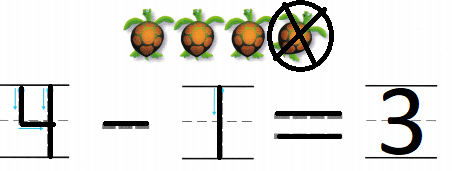Explanation:
There are 4 turtles
1 turtle crab away
4 – 1 = 3
so, 3 turtles are remining.

Question 3.Explanation:
There are 4 scorpions
3 crawl away
4 – 3 = 1
so, 1 scorpion is remaining.

DIRECTIONS: 4-6. Listen to the subtraction word problem. Draw a circle and mark an X to show how many leave. Trace and write to complete the subtraction sentence.

Question 4.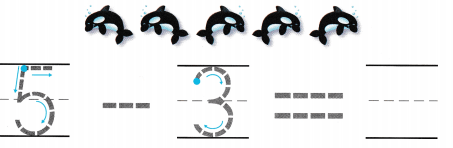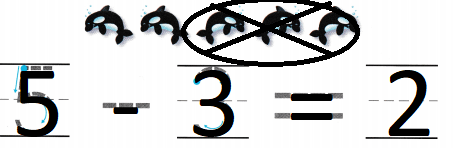Explanation:
There are 5 dolphins
5 – 3 = 2
so, 2 dolphins are there

Question 5.Explanation:
There are 3 fishes
3 – 2 = 1
so, 1 fish is there.

Question 6.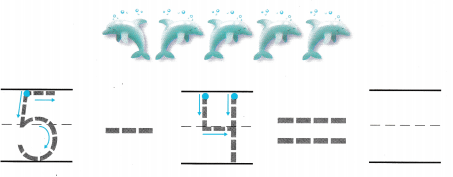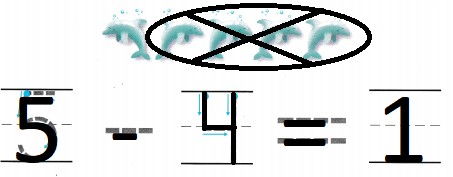Explanation:
There are 5 whales
4 swam away
5 – 4 = 1
so, 1 whale is there.

HOME ACTIVITY • Have your child draw a set of five balloons. Have him or her circle and mark an X on some balloons to show that they have popped. Then have your child write a number sentence to show the subtraction.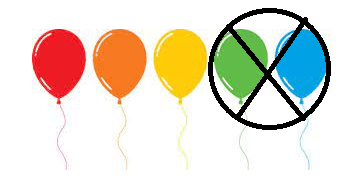Explanation:
There are set of 5 balloons
2 were popped out
5 – 2 = 3
so, three where still blown.

DIRECTIONS: 7. Kristen has four flowers. She gives her friend two flowers. How many flowers does Kristen have now? Draw to solve the problem. Trace and write to complete the subtraction sentence. 8. Choose the correct answer. There are four snails. One snail crawls away. What is 4 – 1?

Problem Solving

Question 7.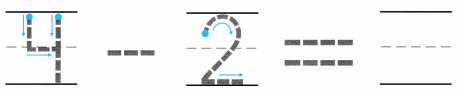Explanation:
Kristen has four flowers.
She gives her friend two flowers.
4 – 2 = 2
so, 2 flowers that Kristen have now

Question 8.Explanation:
There are four snails.
One snail crawls away.
4 – 1 = 3
so, there are 3 snails.

### Texas Go Math Kindergarten Lesson 12.2 Homework and Practice Answer Key

DIRECTIONS: Listen to the subtraction word problem. Circle and mark an X to show how many leave. Trace and write to complete the subtraction sentence. 1. There are four butterflies. Two fly away. How many are left? 2. There are three kittens. One runs away. How many are left?

Question 1.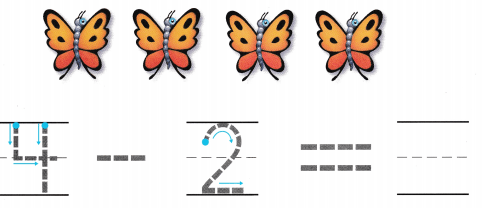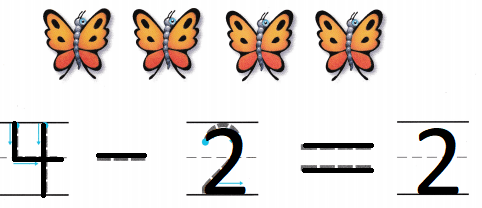Explanation:
There are four butterflies.
Two fly away.
4 – 2 = 2
so, 2 are left

Question 2.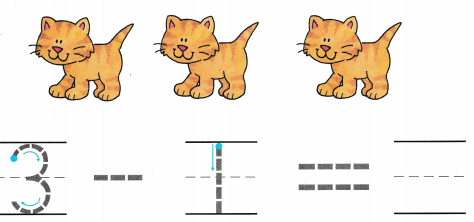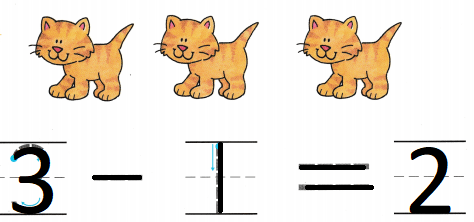Explanation:
There are three kittens.
One runs away.
3 – 1 = 2
so, 2 are left

DIRECTIONS: Choose the correct answer. 3. There are five bears. One bear leaves. What is 5 – 1? 4. There are 4 kittens. Two kittens leave. What is 4 – 2?

Lesson Check

Question 3.Explanation:
There are five bears.
One bear leaves.
5 – 1 = 4
so, there are 4 bears.

Question 4.